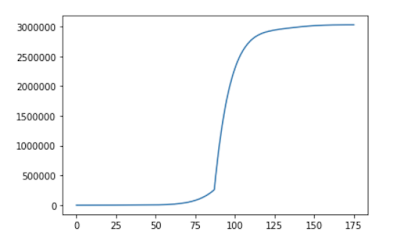top of page
Search

# Forecasting total deaths from CovonavirusAlmost 60, 000 people have died of Coronavirus and we have not reached even the peak of expected distribution of deaths. Expected distribution is somewhat bell shaped curve. Like plot of China's death because of Coronavirus-

Here I have taken machine-learning based approach to forecast total deaths from corona virus.

python( 3.6) code to do analysis-

```  import pandas as pd

url='https://raw.githubusercontent.com/CSSEGISandData/COVID-       19/master/csse_covid_19_data/csse_covid_19_time_series/time_series_covid19_deaths_global.csv'

return(corona_data)```

data is updated daily, so one will get till date information of cases.

Step 2 data preprocessing- visit github page-

Pre Processing code

Step 3 forecasting deaths-

```multiplicative ets model-
fit2_mul = ExponentialSmoothing(plot_dealts1, seasonal_periods=None, trend='mul', seasonal=None).fit(use_boxcox=True)

Step 4- As this is not exact time series analysis. As actual distribution of deaths is bell shaped curve. We need to assume that peak will come in x number of days. Then we calculate number of deaths till peak and just multiply it with 2, to get total deaths.the above plot is expected number of people dies if corona virus takes 15 more days to reach its peak.( additive etc model)

total deaths with additive etc model assuming it will take 10 days to reach its peak- 162996

total deaths with additive etc model assuming it will take 15 days to reach its peak- 280068

total deaths with additive etc model assuming it will take 20 days to reach its peak- 404939

total deaths with multiplicative etc model assuming it will take 10 days to reach its peak- 185368

total deaths with multiplicative etc model assuming it will take 15 days to reach its peak- 335326

total deaths with multiplicative etc model assuming it will take 20 days to reach its peak- 614004

In optimistic scenario, if we get the peak in just 5 day so total deaths would be 99408 with additive and 103785 with multiplicative model. The total death trend will be-Complete code is present at Python Code Corona Virus Deaths

TO know about the Explainable AI- X_AI